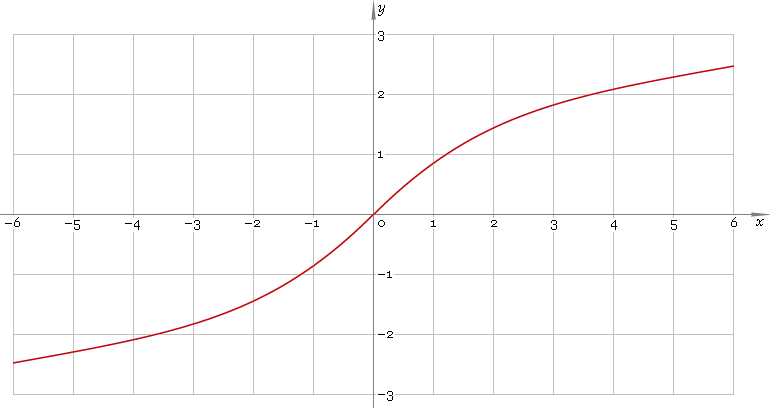The Art of Interface

# arsinh or arsh — arc-hyperbolic sine function

Category. Mathematics.

Abstract. Arc-hyperbolic sine: definition, plot, properties and identities.

## 1. Definition

Arc-hyperbolic sine is inverse of hyperbolic sine function. With the help of natural logarithm it can be represented as:

arsinhx ≡ ln[x + √(x2 + 1)]

## 2. Plot

Arc-hyperbolic sine is antisymmetric function defined everywhere on real axis. Its plot is depicted below — fig. 1.Fig. 1. Plot of the arc-hyperbolic sine function y = arsinhx.

Function codomain is entire real axis.

## 3. Identities

Property of antisymmetry:

arsinh−x = −arsinhx

Reciprocal argument:

arsinh(1/x) = arcschx

Sum and difference:

arsinhx + arsinhy = arsinh[x√(y2 + 1) + y√(x2 + 1)]
arsinhx − arsinhy = arsinh[x√(y2 + 1) − y√(x2 + 1)]
arsinhx + arcoshy = arsinh{xy + √[(x2 + 1)(y2 − 1)]} = arcosh[y√(x2 + 1) + x√(y2 − 1)]

## 4. Support

Arc-hyperbolic sine function arsinh or arsh of the real argument is supported by free version of the Librow calculator.

Arc-hyperbolic sine function arsinh or arsh of the complex argument is supported by professional version of the Librow calculator.

## 5. How to use

To calculate arc-hyperbolic sine of the number:

``arsinh(-1);``

To calculate arc-hyperbolic sine of the current result:

``arsinh(rslt);``

To calculate arc-hyperbolic sine of the number x in memory:

``arsinh(mem[x]);``